### 10.5.1.3 Converting the tree to a single-stage game

Up to this point, solutions have been determined for the alternating-play and the stage-by-stage models. The open-loop model remains. In this case, there is no exchange of information between the players until the game is finished and they receive their costs. Therefore, imagine that players engaged in such a sequential game are equivalently engaged in a large, single-stage game. Recall that a plan under the open-loop model is a function over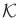. Let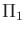and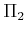represent the sets of possible plans for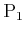and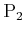, respectively. For the game in Figure 10.13,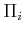is a set of four possible plans for each player, which will be specified in the following order: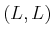,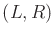,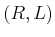, and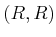. These can be arranged into a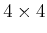matrix game: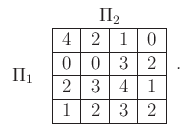(10.105)

This matrix game does not have a deterministic saddle point. Unfortunately, a four-dimensional linear programming problem must be solved to find the randomized value and equilibrium. This is substantially different than the solution obtained for the other two information models.

The matrix-game form can also be derived for sequential games defined under the stage-by-stage model. In this case, however, the space of plans is even larger. For the example in Figure 10.13, there are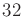possible plans for each player (there are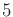decision vertices for each player, at which two different actions can be applied; hence,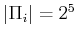for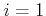and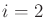). This results in a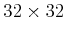matrix game! This game should admit the same saddle point solution that we already determined. The advantage of using the tree representation is that this enormous game was decomposed into many tiny matrix games. By treating the problem stage-by-stage, substantial savings in computation results. This power arises because the dynamic programming principle was implicitly used in the tree-based computation method of decomposing the sequential game into small matrix games. The connection to previous dynamic programming methods will be made clearer in the next section, which considers sequential games that are defined over a state space.

Steven M LaValle 2020-08-14